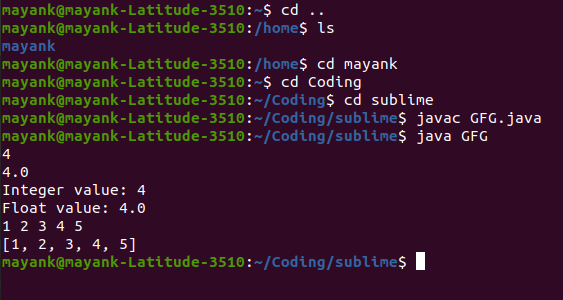# Efficiently Reading Input For Competitive Programming using Java 8

• Difficulty Level : Easy
• Last Updated : 08 Oct, 2021

As we all know, while solving any CP problems, the very first step is collecting input or reading input. A common mistake we all make is spending too much time on writing code and compile-time as well. In Java, it is recommended to use BufferedReader over Scanner to accept input from the user. Why? It is discussed in one of our previous articles here. (Also, the issues associated with the java.util.Scanner is available) Yet for a better understanding, we will go through both the implementations in this article.

• Using Scanner class
• Using BufferedReader class with help of streams (More optimized)

Now let us discuss ways of reading individually to depth by providing clean java programs and perceiving the output generated from the custom input.

Way 1: Simple Scanner Input Reading

The java.util.Scanner class provides inbuilt methods to read primitive data from the console along with the lines of text. In the below code snippet let’s understand how it is done.

Example

## Java

 `// Java Program Illustrating Reading Input``// Using Scanner class` `// Importing Arrays and Scanner class``// from java.util package``import` `java.util.Arrays;``import` `java.util.Scanner;` `// Main class``class` `GFG {` `    ``// Main driver method``    ``public` `static` `void` `main(String[] args)``    ``{``        ``// Creating object of Scanner class``        ``Scanner scan = ``new` `Scanner(System.in);` `        ``// Basic Input Reading``        ``int` `a = scan.nextInt();``        ``float` `b = scan.nextFloat();` `        ``System.out.println(``"Integer value: "` `+ a);``        ``System.out.println(``"Float value: "` `+ b);` `        ``// Space Separated Input Reading``        ``int``[] arr = ``new` `int``[``5``];` `        ``for` `(``int` `i = ``0``; i < arr.length; i++) {``            ``arr[i] = scan.nextInt();``        ``}` `        ``System.out.println(Arrays.toString(arr));``    ``}``}`

Output:From the above Linux shell output we can conclude that input is given as is follows:

```4
5.6
1 2 3 4 5```

The output generated is as follows:

```Integer value: 4
Float value: 5.6
[1, 2, 3, 4, 5]```

The above example illustrates the most common approach used by the majority of programmers while solving competitive programming problems. But what if we can enhance our code a bit to make it faster and reliable?

java.io.BufferedReader class does not provide any method to read primitive data inputs. Java.io.BufferedReader class reads text from a character-input stream, buffering characters so as to provide for the efficient reading of the sequence of characters. Although it throws a checked exception known as IOException. Let us see how to handle that exception and read input from the user. Consider custom input as below as follows:

Input:

```4
5.6
1 2 3 4 5```

Example

## Java

 `// Java Program Illustrating Reading Input``// Using` `// Importing required classes``import` `java.io.BufferedReader;``import` `java.io.IOException;``import` `java.io.InputStreamReader;``import` `java.util.Arrays;` `// Main class``class` `GFG {` `    ``// Main driver method``    ``public` `static` `void` `main(String[] args)``        ``throws` `IOException``    ``{` `        ``// Reading input via BufferedReader class``        ``BufferedReader br = ``new` `BufferedReader(``            ``new` `InputStreamReader(System.in));` `        ``// Basic Input Reading``        ``int` `a = Integer.parseInt(br.readLine());``        ``float` `b = Float.parseFloat(br.readLine());` `        ``// Print above input values in console``        ``System.out.println(``"Integer value: "` `+ a);``        ``System.out.println(``"Float value: "` `+ b);` `        ``// Space Separated Input Reading``        ``int``[] arr = ``new` `int``[``5``];``        ``String[] strArr = br.readLine().split(``" "``);` `        ``for` `(``int` `i = ``0``; i < arr.length; i++) {``            ``arr[i] = Integer.parseInt(strArr[i]);``        ``}` `        ``// Printing the elements in array``        ``// using toString() method``        ``System.out.println(Arrays.toString(arr));``    ``}``}`

Output:

```Integer value: 4
Float value: 5.6
[1, 2, 3, 4, 5]```

The above example illustrates another common approach used to read the data while solving competitive programming problems. So is this enough? What if we can enhance it even more? Yes. It is possible. Stay tuned.

Method 3: Enhanced way for reading separated data using BufferedReader via Streams

In the previous examples, we have seen while reading space-separated data we stored it first in a String array, and then we iterated over elements and then used java typecasting to convert it to the required data type. How about a single line of code making this possible? Yes. Java 8’s stream library provides a variety of functions to make it easy and optimized. Consider custom input as below as follows:

Input:

```34 55 78 43 78 43 22
94 67 96 32 79 6 33```

Example

## Java

 `// Java Program to Read Separated Data``// Using BufferedReader class voa enhanced for loopd` `// Importing required classes``import` `java.io.BufferedReader;``import` `java.io.IOException;``import` `java.io.InputStreamReader;``import` `java.util.Arrays;``import` `java.util.List;``import` `java.util.stream.Collectors;``import` `java.util.stream.Stream;` `// Main class``class` `GFG {` `    ``// Main driver method``    ``public` `static` `void` `main(String[] args)``        ``throws` `IOException``    ``{` `        ``// Reading input separated by space``        ``BufferedReader br = ``new` `BufferedReader(``            ``new` `InputStreamReader(System.in));` `        ``// Storing in array``        ``int``[] arr = Stream.of(br.readLine().split(``" "``))``                        ``.mapToInt(Integer::parseInt)``                        ``.toArray();``        ``System.out.println(Arrays.toString(arr));` `        ``// Using streams concepts to parse map to integer``        ``// later on collecting via Collectors via toList()``        ``// method and storing it an integer list``        ``List arrayList``            ``= Stream.of(br.readLine().split(``" "``))``                  ``.mapToInt(Integer::parseInt)``                  ``.boxed()``                  ``.collect(Collectors.toList());` `        ``// Print the above List as created``        ``System.out.println(arrayList);``    ``}``}`

Output:

```[34, 55, 78, 43, 78, 43, 22]
[94, 67, 96, 32, 79, 6, 33]```

The above example illustrates how we can read separated input and store it into the required data structure using a single line of code. Using java8 there might be a possibility that programmers are comfortable with List collection. That’s why it is covered. Now, let us understand code word by word.

Storing into int array:

1.  java.util.stream.Stream.of() – Creates stream of string array passed

2. br.readLine().split(” “) – Converts input string into string array based on separator value. (Blank Space – ” ” in example)

3. mapToInt(Integer::parseInt) – Converts String element into the  required data type using suitable mapper function (Integer’s  parseInt() in example)

4. toArray() – converts the stream of  int elements into an array

Storing into the List Collection:

1. java.util.stream.Stream.of() – Creates stream of string array passed

2. br.readLine().split(” “) – Converts input string into string array based on separator value. (Blank Space – ” ” in example)

3. mapToInt(Integer::parseInt) – Converts String element into the  required data type using suitable mapper function (Integer’s  parseInt() in example)

4. boxed() – boxes the stream to Integer elements

5. collect(Collectors.toList()) – creates a collection of Integer elements and converts it to the java.util.List Collection.

My Personal Notes arrow_drop_up